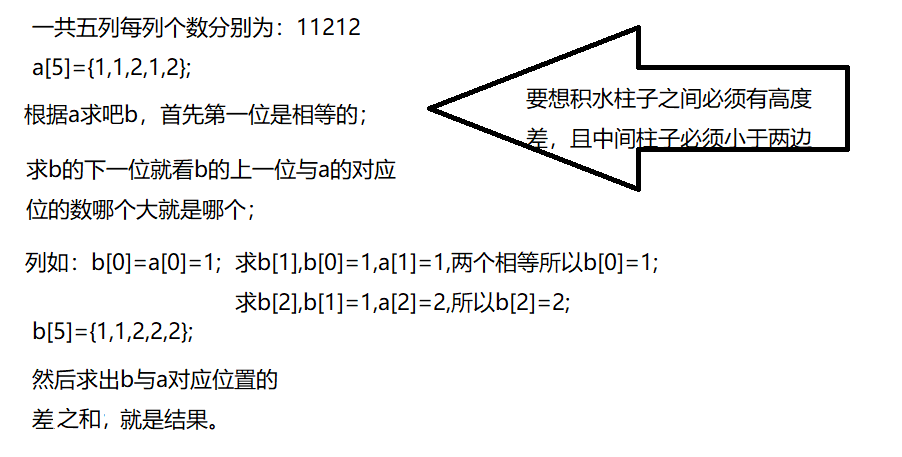2021-08-05 21:50

# c语言求积水面积，整不会了啊

11212

• 好问题 提建议
• 收藏

#### 3条回答默认 最新

•Pink_Piggy 2021-08-05 23:01
已采纳``````
#include<stdio.h>

int main()
{
int n;
int result = 0;

printf("请输入有几列立方体：");
scanf_s("%d", &n, 4);

int* a = new int[n];
int* b = new int[n];
printf("分别输入每列立方体的个数:");
for (int i = 0; i < n; ++i)
{
scanf_s("%d", &a[i], 4);
}

b = a;
for (int i = 1; i <= n-1; ++i)
{
if (a[i] < b[i - 1])
b[i] = b[i - 1];
else
b[i] = a[i];
}

for (int i = 0; i < n; i++)
{
result += b[i] - a[i];
}

printf("%d", result);

delete a;
delete b;

return 0;
}
``````

这是我的理解哦，如果有错误请帮忙指出！

已采纳该答案
评论
解决 1 无用
打赏 举报
•大笨比一个 2021-08-05 22:18

双指针可以解决，考虑只有0的地方可以积水

评论
解决 无用
打赏 举报
•英雄哪里出来 2021-08-05 22:38
``````
#define ll long long

class Solution {
public:
long long maxWater(vector<int>& arr) {
stack <int> st;
ll ans = 0;
for(int i = 0; i < arr.size(); ++i) {
if(st.empty() || arr[i] < arr[ st.top() ]) {
st.push(i);                                                                   //   (1) 维护一个单调递减栈
}else {
int h = i;
while(!st.empty() && arr[i] >= arr[ st.top() ]) {            //   (2) 当前元素 >= 栈顶
if(st.size() == 1) {
st.pop();
h = i;                                                                 //   (3)  栈为 1 直接跳出
break;
}
h = st.top();
st.pop();
int dh = min(arr[i], arr[st.top()]) - arr[h];               // (4) 能够盛水的最大高度
int dw = i - h;                                                       // (5) 能够盛水的最大宽度
ans += (ll)dw * dh;                                               // (6) 相乘就是面积
arr[h] = arr[i];                                                       // (7) 这一步是为了削峰
}
st.push(h);

}
}
return ans;
}
};
``````
评论
解决 无用
打赏 举报